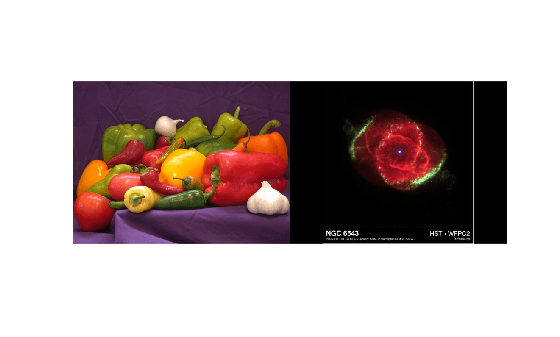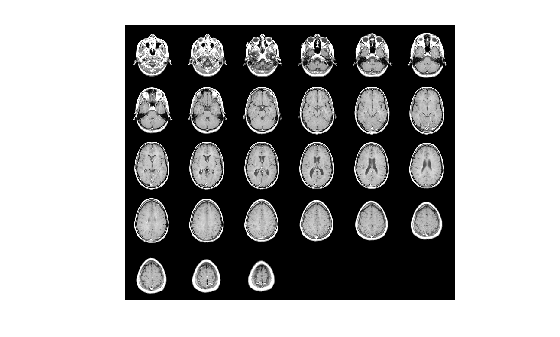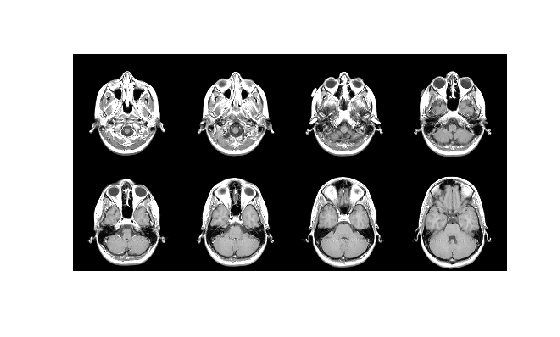# imtile

Combine multiple image frames into one rectangular tiled image

## Syntax

``out = imtile(filenames)``
``out = imtile(I)``
``out = imtile(images)``
``out = imtile(imds)``
``out = imtile(___,map)``
``out = imtile(___,Name,Value)``

## Description

``` `out = imtile(filenames)` returns a tiled image containing the images in the files with file names `filenames`.By default, the `imtile` function arranges the images so that they roughly form a square. You can change the arrangement using optional name-value arguments. The images can have different sizes and data types.If you specify an indexed image, then the `imtile` function converts it to RGB using the colormap present in the file.If there is a data type mismatch between images, then the `imtile` function converts all images to data type `double` using the `im2double` function.```
````out = imtile(I)` returns a tiled image containing all the frames of the multiframe image array `I`. A multiframe image array can be a sequence of binary, grayscale, or truecolor images.```
``` `out = imtile(images)` returns a tiled image containing the images specified in the cell array `images`. `imtile` displays empty cell array elements as a blank tile.```
``` `out = imtile(imds)` returns a tiled image containing the images specified in the ImageDatastore object `imds`. For information about image datastores, see `ImageDatastore`. ```
````out = imtile(___,map)` returns a tiled image in which grayscale, indexed, and binary images are converted to RGB using the colormap `map`. You can specify input images using the input argument of any of the preceding syntaxes. Note that if you specify images using file names `filenames`, and also specify the colormap, then `map` overrides any internal colormap present in the image files.```
````out = imtile(___,Name,Value)` returns a customized tiled image, depending on the values of the optional parameter name-value pair arguments.```

## Examples

collapse all

Read multiple images from files into the workspace and create a tiled image containing the images. Display the tiled image.

```out = imtile({'peppers.png', 'ngc6543a.jpg'}); imshow(out);```Using a data set containing multiple images, tile the images in a grid.

```load mri out = imtile(D, map); imshow(out);```Create a tiled image containing only the first eight images in the data set. Use the `'GridSize'` parameter to arrange the images in a 2-by-4 grid.

```out = imtile(D, map, 'Frames', 1:8, 'GridSize', [2 4]); figure; imshow(out);```Read an RGB image into the workspace.

`imRGB = imread('peppers.png');`

Create a tiled image containing each of the three planes of the RGB image. Display the tiled image.

```out = imtile(imRGB); imshow(out)```From an image datastore, create and customize a tiled image.

Create an image datastore containing all the files with the file extension `'tif'` or `'png'` in the specified folder.

```fileFolder = fullfile(matlabroot,'toolbox','matlab','imagesci'); imds = imageDatastore(fileFolder,'FileExtensions',{'.tif','.png'});```

Create a tiled image containing the images in the datastore.

```out1 = imtile(imds); imshow(out1);```Use the `'BorderSize'` and '`BackgroundColor'` parameters to add a blue border to the tiled image.

```out2 = imtile(imds, 'BorderSize', 10, 'BackgroundColor', 'b'); figure; imshow(out2);```## Input Arguments

collapse all

Names of files containing images, specified as an f-by-1 string array, a 1-by-f string array, a character vector, or a cell array of character vectors. If the files are not in the current folder or in a folder on the MATLAB® path, then specify the full path name. For more information, see `imread`.

Example: `'file1.jpg'`

Example: `["file1.jpg" "file2.jpg"]`

Example: `'../dir/data/file1.png'`

Example: `{'C:\dir\data\file1.tif','C:\dir\data\file2.tif'}`

Data Types: `char` | `string` | `cell`

Multiframe image, specified as a numeric array. `I` can be:

• An m-by-n-by-k array representing a sequence of k binary, grayscale, or indexed images

• An m-by-n-by-1-by-k array representing a sequence of k binary, grayscale, or indexed images

• An m-by-n-by-3-by-k array representing a sequence of k truecolor images

List of images, specified as an k-by-1 or 1-by-k cell array of numeric matrices. The cell array can contain numeric matrices of size m-by-n or m-by-n-by-3.

Image datastore, specified as an `ImageDatastore` object.

Colormap, specified as a c-by-3 numeric matrix with values in the range [0,1]. Each row of `map` is a three-element RGB triplet that specifies the red, green, and blue components of a single color. When you specify `map`, the `imtile` function converts all indexed, grayscale, and binary images to truecolor using this colormap.

Data Types: `double`

### Name-Value Arguments

Specify optional pairs of arguments as `Name1=Value1,...,NameN=ValueN`, where `Name` is the argument name and `Value` is the corresponding value. Name-value arguments must appear after other arguments, but the order of the pairs does not matter.

Before R2021a, use commas to separate each name and value, and enclose `Name` in quotes.

Example: ```imtile({'peppers.png','ngc6543a.jpg'},'BackgroundColor','g'); ```

Color of the background, specified as an RGB triplet, a color name, or a short color name. The `imtile` function fills all blank spaces with this color, including the space specified by `'BorderSize'`.

You can specify any color using an RGB triplet. An RGB triplet is a 3-element row vector whose elements specify the intensities of the red, green, and blue components of the color. The intensities must be in the range [0,1].

You can specify some common colors by name as a string scalar or character vector. This table lists the named color options and the equivalent RGB triplets.

Color NameShort NameRGB TripletAppearance
`'red'``'r'``[1 0 0]``'green'``'g'``[0 1 0]``'blue'``'b'``[0 0 1]``'cyan'` `'c'``[0 1 1]``'magenta'``'m'``[1 0 1]``'yellow'``'y'``[1 1 0]``'black'``'k'``[0 0 0]``'white'``'w'``[1 1 1]`Example: `'BackgroundColor','r'`

Example: `'BackgroundColor','green'`

Example: `'BackgroundColor',[0 0.4470 0.7410]`

Padding around each thumbnail image, specified as the comma-separated pair consisting of `'BorderSize'` and a numeric scalar or 1-by-2 numeric vector of the form `[brows bcols]`. The `imtile` function pads the borders of each image with the background color.

Frames to include, specified as the comma-separated pair consisting of `'Frames'` and a numeric array or a logical mask. The `imtile` function interprets the values as indices into the image array or cell array. The following examples create a tiled image containing the first three image frames.

Example: `out = imtile(I,'Frames',1:3);`

Example: ```out = imtile(I,'Frames',[true true true]);```

Number of rows and columns of thumbnails in the tiled image, specified as the comma-separated pair consisting of `'GridSize'` and a two-element vector of the form `[nrows ncols]`. `nrows` specifies the number of rows in the grid and `ncols` specifies the number of columns in the grid. Use `NaN`s or `Inf`s to have `imtile` calculate the size in a particular dimension in a way that includes all the images.

• If `'GridSize'` is `[2 NaN]`, then `imtile` creates a tiled image with two rows and the number of columns necessary to include all the images.

• If both the elements are `NaN` or `Inf`, then `imtile` calculates the grid size to form a square. `imtile` returns the images horizontally across columns.

• If there is a mismatch between `GridSize` and number of images (frames), then `imtile` creates the tiled image based on `GridSize`.

Size of thumbnails, specified as the comma-separated pair consisting of `'ThumbnailSize'` and a two-element vector of the form `[trows tcols]`, in pixels. The `imtile` function preserves the aspect ratio of the original image by zero-padding the boundary.

• If you specify a `NaN` or `Inf`, then the `imtile` function calculates the corresponding value automatically to preserve the aspect ratio of the first image.

• If you specify an empty array (`[]`), then the `imtile` function uses the full size of the first image as the thumbnail size.

## Output Arguments

collapse all

Tiled output image, returned as a numeric matrix or a numeric array. The output image is an M-by-N-by-3 numeric array when any of these conditions are true:

• At least one of the input images is an RGB image or indexed image

• You specify a colormap, `map`

• You specify a background color, `'BackgroundColor'`, regardless of whether the tiled image includes background pixels or not

## Version History

Introduced in R2018b